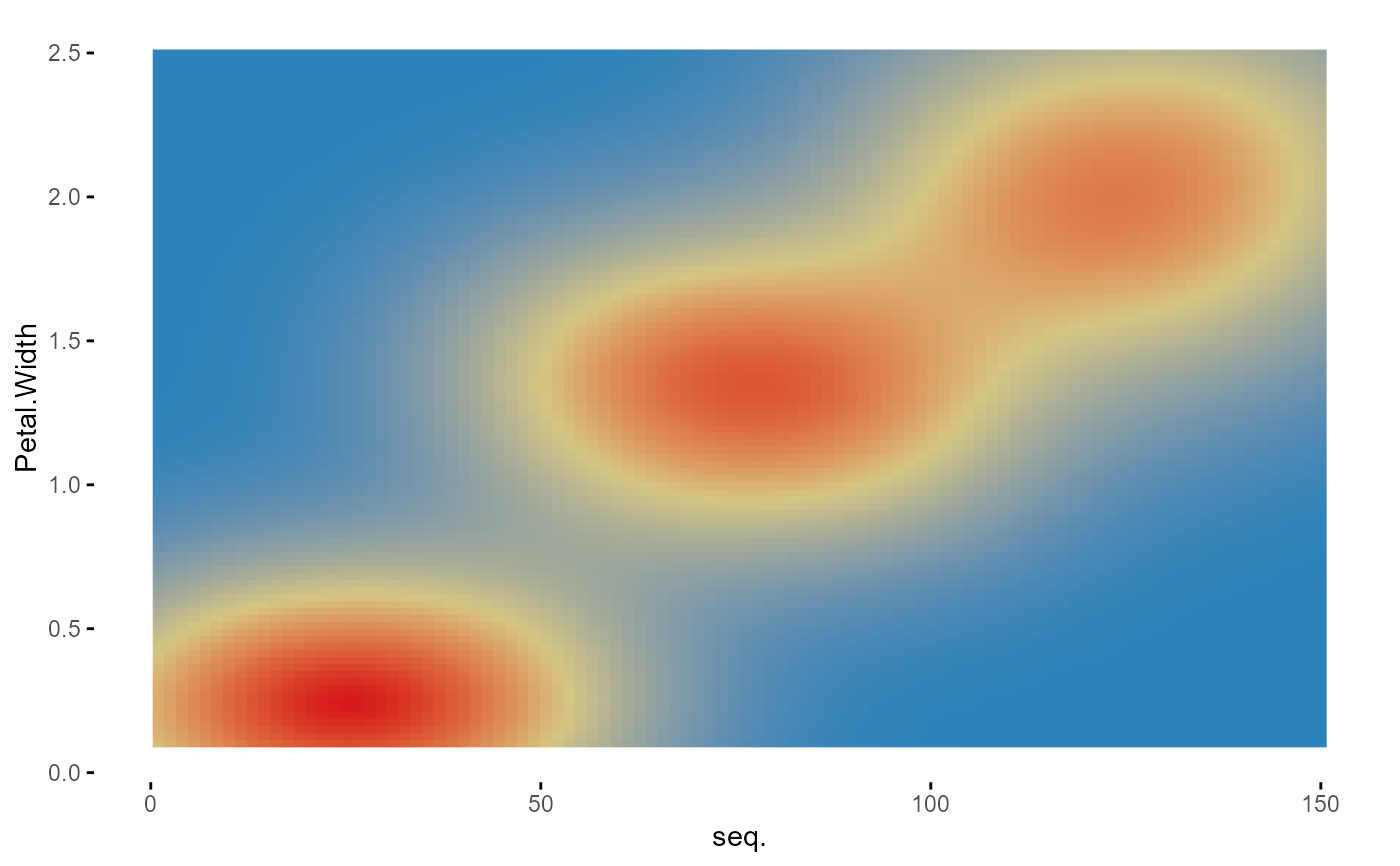In order to present the graphic, the user must define a dataset, at least one variable whitin this dataset and a compatible type of graphic. Future work will include graphics that can combine up to three variables.

plotup(data, vars, diagram, output = "plots pane", dir = tempdir())

## Arguments

data Data.frame. Default dataset to use for plot. If not already a data.frame, it should be first coerced to by [as.data.frame()]. Character. A variable within the dataset. Character. A specific graphic to be presented within the ones considered by the 'logical', 'ordered', 'factor', 'character', 'datetime' and 'numeric' arguments of the 'wideplot()' function. Character. Type of output. 'html': Creates and displays a html file with the specific graphic. 'plots pane': Default output, a ggplot2 object in RStudio's plots pane. 'console': Prints the code that produces the specific graphic. Directory in which the files are stored.

## Value

This function returns a c('gg', 'ggplot') object, but if the 'output' argument is set to it 'html' or 'console', the function cause a side-effect: either creating and displaying a temporary html file, or printing the ggplot2 code to the console.

## Examples

plotup(iris, "Petal.Width", "color heatmap")plotup(iris, "Petal.Width", "color heatmap", output = "console")
#>
#> ggplot(iris, aes(y=Petal.Width)) +
#>   stat_density_2d(aes(x=seq_along(Petal.Width), fill = stat(density)), geom = 'raster', contour = FALSE) +
#>   scale_fill_gradientn(colours = colorRampPalette(rev(RColorBrewer::brewer.pal(4, 'Spectral')))(3)) +
#>   labs(x='seq.') +
#>   theme_minimal() +
#>   theme(panel.grid = element_line(colour = NA),
#>     axis.ticks = element_line(color = 'black'),
#>     legend.position='none')if (interactive()) {
plotup(iris, "Petal.Width", "color heatmap", output = "html")
}